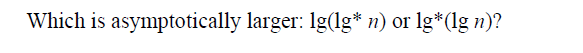##### Which is asymtotically larger?##### 1Comment
shivani
18 Aug 2017 04:48 pm

log*n denotes iterated logarithm , it means apply log any no. of times until you get 1.

The iterated logarithm is a very slowly growing function:

lg∗2=1lg∗⁡2=1,

lg∗4=2lg∗⁡4=2,

lg∗16=3lg∗⁡16=3,

lg∗65536=4lg∗⁡65536=4,

lg∗265536=5lg∗⁡265536=5.
So, coming to question lg(lg* n) and lg*(lg n)
lg(lg* n) can be written as lg(c) , where c is some constant
and , lg*(lg n) will be 1
so, lg(lg* n) is asymptotically larger than lg*(lg n)